# Mechanical Energy – Examples, PDF

Often, we overlook the various physics-based mechanisms that come into play in our everyday lives and actions. One of these common physics-based mechanisms is mechanical energy.

## 1. Conservation of Mechanical energy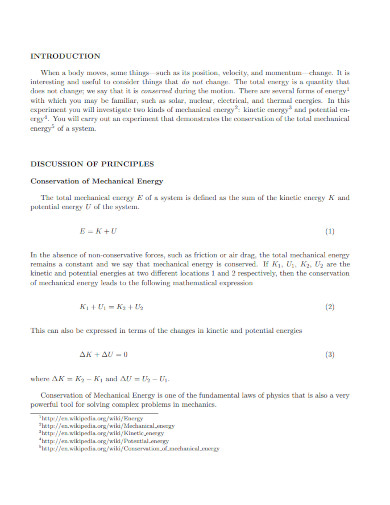webassign.net

## 2. Mechanical Energy  Moving Experience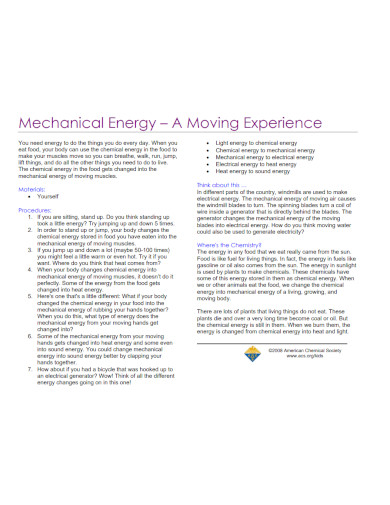acs.org

## 3. Mechanical Energy Storage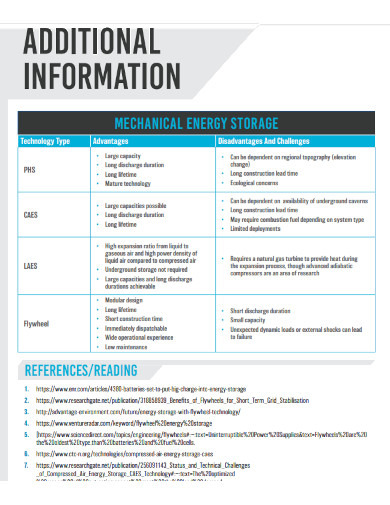netl.doe.gov

## 4. Electro Mechanical Energy Conversion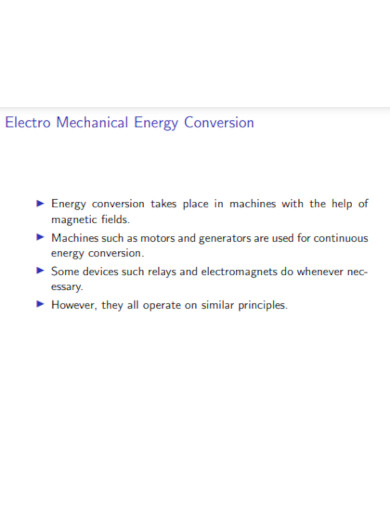iitp.ac.in

## 5. Development of Mechanical Energy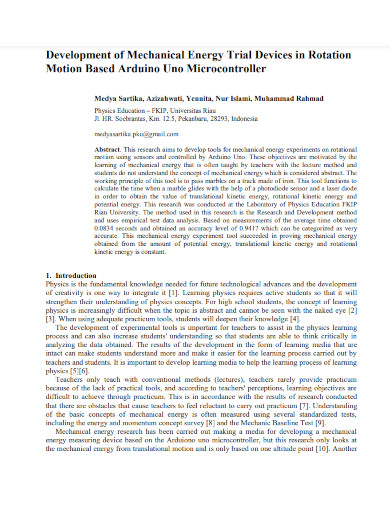iopscience.iop.org

## 6. Phone Charging Using Human Mechanical Energy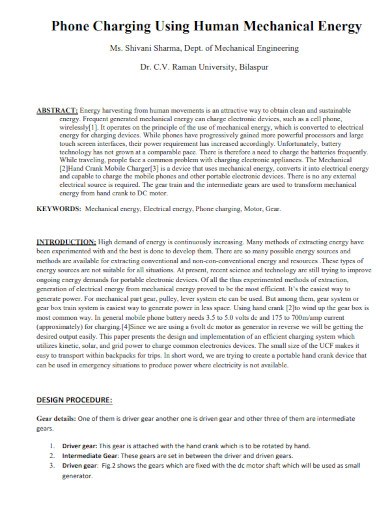pramanaresearch.org

## 7. Recent Progress on Bio-mechanical Energy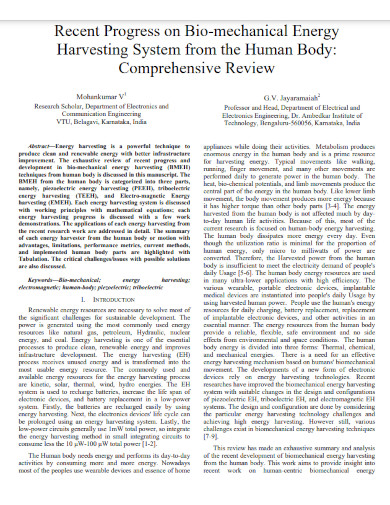thesai.org

## 8. Converting Mechanical Energy into Heat Energyld-didactic.de

## 9. Electromechanical Energy Conversion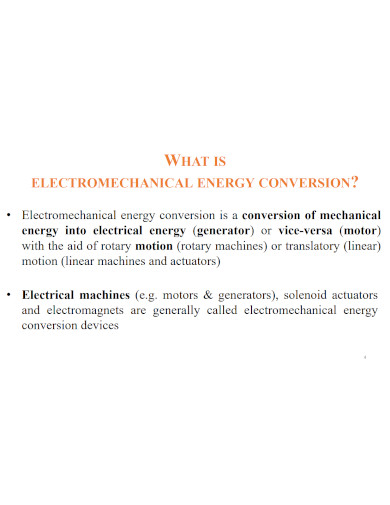web.iitd.ac.in

## 10. Heat Equivalent of Mechanical Energy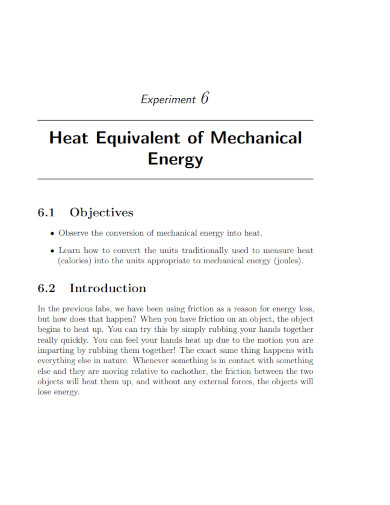web.pa.msu.edu

## What Is Mechanical Energy

Mechanical energy is the energy an object possesses in the context of motion and work. Not only that but mechanical energy is the summation of the potential and kinetic energy of an object at work. This means that mechanical energy is the energy exerted when a specific entity or object is at work.

## How to Calculate Mechanical Energy

Mechanical energy follows a very simple formula of (M.E.) = ((1/2)mv^2) + (m × g × h). Where M.E. is mechanical energy (J), m is the mass of the object (kg), v is the velocity of the object (ms^-1), g is the acceleration due to gravity (constant 9.81 m/s^2), and h is the height of the object (m).

### Step 1: Write Down the Formula for Mechanical Energy

Begin by writing down the formula for mechanical energy, so that you have a visual outline of the final form of the equation. The formula should be (M.E.) = ((1/2)mv^2) + (m × g × h).

### Step 2: List Down the Given Variables and Ensure They Have Correct Measurements

After you have written the formula for mechanical energy, you will need to list down all the variables given in the problem. Doing this will help you reduce instances of confusion when substituting the values. Not only that but you have to ensure each variable has the correct applicable measurement. If any of the variables are the wrong measurement, convert them accordingly.

### Step 3: Substitute the Values and Solve the Equation

You must substitute each variable to the correct value in the mechanical energy equation. After doing that, you will be presented with a missing variable which the equation will try to answer.

### Step 4: Ensure That the Answered Value Has The Correct Measurement

When you have solved the equation, you must ensure that the value you answered is the correct measurement value. If the variable you have obtained from the mechanical energy equation is not the asked measurement, convert it accordingly.

## What is the difference between kinetic energy and mechanical energy?

People have defined kinetic energy as the energy exerted by an object in motion, which is the juxtaposition of potential energy. An object exerts kinetic energy through actions that involve work and movement. Mechanical energy, on the other hand, is the overarching energy that kinetic and potential are categorized into. This energy refers to both the potential energy and possible kinetic energy a single object can create and manipulate. Mechanical energy is the system that takes into account the potential energy of an object in place and the kinetic energy of an object put to work. In conclusion, kinetic energy deals with a specific part of work, whilst mechanical energy takes into account the whole system.

## What happens to an object when mechanical energy is applied?

Following Newton’s third law of motion, wherein Newton states that every object has an equal and opposite reaction, the object exerted upon will move or stay still depending on the exerted mechanical force and energy. This means that an object at work will either push or pull an object of lesser resistance to the direction the moving object is directed at. If the inertia is stronger than the force of the working object, then said object will not move or displace the other object it has impacted.

## How does the concept of mechanical energy connect with electrical energy?

Mechanical energy is one of the most important and abundant forms of energy that one can easily observe in nature. This makes it very easy for people to utilize and harvest electrical energy through the usage of specialized tools and instruments. The reason why people can do this is because of the law of conservation of energy, which states that energy can never be created nor destroyed, only converted from one form to another. Using this principle, humans have been able to convert mechanical energy into electrical energy through the use of hydropower plants and wind turbines.

Mechanical energy is a type of energy that deals with the motion of an object through the concept of work. This type of energy is very abundant and can be converted into heat or electrical energy, which is used in both exercise and electrical generation. Therefore, it is very important to understand the concept of mechanical energy, even a simple grasp of this concept allows one to know how this energy works and affects our everyday lives.